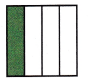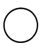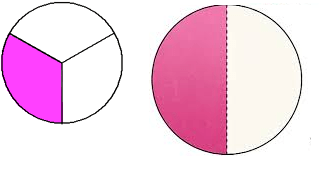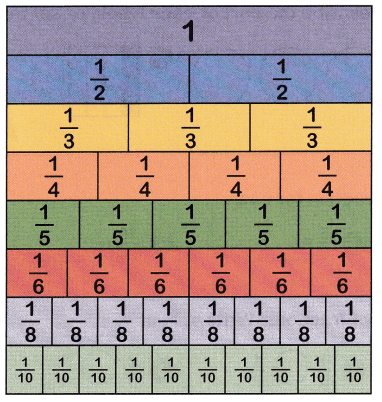Practice the questions of McGraw Hill Math Grade 4 Answer Key PDF Chapter 7 Test to secure good marks & knowledge in the exams.

Write a fraction to describe the part of each whole or set that is green.

Question 1.$$\frac{2}{5}$$
Explanation:
There are 5 circles out of it 2 are colored
2 out of 5 are colored.

Question 2.$$\frac{1}{4}$$
Explanation:
There are 4 boxes out of them 1 is colored
1 out of 4 is colored

Find an equivalent fraction for each.

Question 3.
$$\frac{1}{4}$$ = ____
$$\frac{1}{4}$$ = $$\frac{2}{8}$$
Explanation:
The equivalent fraction of $$\frac{2}{8}$$  is $$\frac{1}{4}$$
2 x 1 = 2
2 x 4 = 8
Multiplying numerator and denominator by 2

Question 4.
$$\frac{5}{6}$$ = ____
$$\frac{5}{6}$$ = $$\frac{10}{12}$$
Explanation:
The equivalent fraction of $$\frac{5}{6}$$ is $$\frac{10}{12}$$
5 x 2 = 10
6 x 2 = 12
Multiplying numerator and denominator by 2

Question 5.
$$\frac{16}{24}$$ = ____
$$\frac{16}{24}$$ = $$\frac{2}{3}$$
Explanation:
The equivalent fraction of $$\frac{16}{24}$$ is $$\frac{2}{3}$$
Dividing the numerator and denominator by 8
8 x 2 = 16
8 x 3 = 24

Question 6.
$$\frac{10}{20}$$ = ____
$$\frac{10}{20}$$ = $$\frac{1}{2}$$
Explanation:
The equivalent fraction of $$\frac{10}{20}$$ is $$\frac{10}{20}$$
Dividing the numerator and denominator by 10
10 x 1 = 10
10 x 2 = 20

Compare each pair of fractions. Write <,>, or =.

Question 7.
$$\frac{1}{4}$$$$\frac{2}{3}$$
$$\frac{1}{4}$$$$\frac{2}{3}$$
Explanation:
$$\frac{1}{4}$$ is lesser than the $$\frac{2}{3}$$
so, represented with lesser than symbol

Question 8.
$$\frac{4}{8}$$$$\frac{1}{2}$$
$$\frac{4}{8}$$$$\frac{1}{2}$$
Explanation:
$$\frac{4}{8}$$ is equal to $$\frac{1}{2}$$
so, represented with equal symbol

Question 9.
$$\frac{2}{5}$$$$\frac{1}{3}$$
$$\frac{2}{5}$$$$\frac{1}{3}$$
Explanation:
$$\frac{2}{5}$$ is greater than  $$\frac{1}{3}$$
so, represented with greater than symbol.

Question 10.
$$\frac{3}{4}$$$$\frac{7}{8}$$
$$\frac{3}{4}$$$$\frac{7}{8}$$
Explanation:
$$\frac{3}{4}$$ is lesser than the $$\frac{7}{8}$$
so, represented with lesser than symbol

Solve.

Question 11.
Henry paints a square piece of wood as shown. He says he painted $$\frac{3}{4}$$ of the board yellow. Is he correct? Why or why not?Yes, he is correct
Explanation:
The square is divided into 4 parts equally
he colored 3 parts as yellow
so, he painted $$\frac{3}{4}$$ of the board yellow

Question 12.
Draw a picture that correctly shows $$\frac{1}{3}$$ < $$\frac{1}{2}$$.Explanation:
According to the pictures
if we shade one third the shaded part is lesser than the
one halves of the shaded parts

Question 13.
Natalie buys 25 buttons. $$\frac{1}{5}$$ of them are brown. $$\frac{2}{5}$$ of them are black. $$\frac{2}{5}$$ of them are gray. How many black buttons are in the bag?
_______ black buttons
10 black buttons
Explanation:
=25($$\frac{2}{5}$$)
= ($$\frac{25}{1}$$)($$\frac{2}{5}$$)
= ($$\frac{50}{5}$$)
=10

Question 14.
There are 24 cans of soup on a shelf. chicken soup. $$\frac{1}{4}$$ are beef soup. $$\frac{1}{4}$$ are tomato soup. How many cans of tomato soup are there?
_______ cans
6 cans of tomato soup
Explanation:
=24($$\frac{1}{4}$$)
= ($$\frac{24}{1}$$)($$\frac{1}{4}$$)
= ($$\frac{24}{4}$$)
=6Use the fraction strips to identify equivalent fractions.

Question 15.
Place a check mark next to the fraction that is equivalent to $$\frac{2}{10}$$.
____ $$\frac{1}{5}$$ ____ $$\frac{3}{10}$$ ____ $$\frac{1}{4}$$
$$\frac{1}{5}$$
Explanation:
When the numerator and denominator are divided by 2
2 x 1 = 2
2 x 5 = 10
$$\frac{2}{10}$$ equivalent fraction is $$\frac{1}{5}$$

Question 16.
Place a check mark next to the fraction that is equivalent to $$\frac{1}{3}$$.
____ $$\frac{4}{8}$$ ____ $$\frac{1}{2}$$ ____ $$\frac{2}{6}$$
$$\frac{2}{6}$$
Explanation:
When the numerator and denominator are multiplied by 2
1 x 2 = 2
3 x 2 = 6
$$\frac{1}{3}$$ equivalent fraction is $$\frac{2}{6}$$

Question 17.
Place a check mark next to the fraction that is equivalent to $$\frac{6}{8}$$.
____ $$\frac{9}{10}$$ ____ $$\frac{3}{4}$$ ____ $$\frac{4}{5}$$
$$\frac{3}{4}$$
Explanation:
When the numerator and denominator are divided by 2
$$\frac{6}{8}$$ is equivalent to $$\frac{3}{4}$$
3 x 2 = 6
4 x 2 = 8

Question 18.
Place a check mark next to the fraction that is equivalent to $$\frac{1}{2}$$.
____ $$\frac{2}{3}$$ ____ $$\frac{3}{8}$$ ____ $$\frac{3}{6}$$
$$\frac{3}{6}$$
Explanation:
the numerator and denominator are multiplied by 3
$$\frac{1}{2}$$ is equivalent to $$\frac{3}{6}$$
1 x 3 = 3
2 x 3 = 6

Compare each pair of fractions. Write <, >, or . Use the fraction strips and $$\frac{1}{2}$$ or $$\frac{1}{4}$$ as benchmarks.

Question 19.
$$\frac{2}{3}$$$$\frac{4}{8}$$
$$\frac{2}{3}$$$$\frac{4}{8}$$
Explanation:
$$\frac{2}{3}$$ is greater than $$\frac{4}{8}$$
represented with greater than symbol

Question 20.
$$\frac{4}{5}$$$$\frac{3}{4}$$
$$\frac{4}{5}$$$$\frac{3}{4}$$
Explanation:
$$\frac{4}{5}$$ is greater than $$\frac{3}{4}$$
represented with greater than symbol

Question 21.
$$\frac{1}{5}$$$$\frac{2}{10}$$
$$\frac{1}{5}$$$$\frac{2}{10}$$